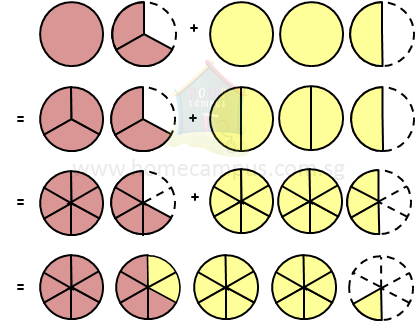## How to Add Mixed Numbers and Fractions?

#### What is a mixed number?

A mixed number or mixed fraction has two parts: a whole number and a proper fraction.

Mixed number  =  whole number  +  proper fraction

#### 2. How many pizzas do I have altogether if I have   1 2 3   pepperoni pizzas and   2 1 2   cheese pizzas?

Pepperoni pizza  =>
 1 2 3

Cheese pizza  =>
 2 1 2

Total  =>
 1 2 3
+
 2 1 21 2 3
+
 2 1 2

 (1 x 3) + 2 3
+
 (2 x 2) + 1 2
(First, change both mixed numbers to improper fractions)
 5 3
+
 5 2

 5 x 2 3 x 2
+
 5 x 3 2 x 3
(Second, change the fractions to like fractions)
 10 6
+
 15 6

 25 6
=  4  +
 1 6
(Finally, change the improper fraction to a mixed number)
 4 1 6

I have
 4 1 6
pizzas altogether.

#### 3. What is    3 1 4  +  1 2 ?

 3 1 4
+
 1 2

 (3 x 4) + 1 4
+
 1 2
(First, change both mixed numbers to improper fractions)
 13 4
+
 1 2

 13 4
+
 1 x 2 2 x 2
(Second, change the fractions to like fractions)
 13 4
+
 2 4

 13 + 2 4